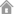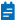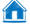当前位置： 首页 > 常见问题 > 站帮主CMS问题 >

***********************站帮主CMS电脑手机仿站之首页标签******************************

<title>{标题}</title>
<meta name="keywords" content="{关键词}"/>
<meta name="description" content="{摘要}"/>

CSS和JS路径:
{样式路径}/css/
{样式路径}/images/
style.css" rel="stylesheet"  type="text/css" />

{包含=top.html}
{包含=left.html}
{包含=foot.html}

<a href="{首页}" class='{首页样式=on}' >网站首页</a>
{导航：编号=全部|条=全部}
<a href="[链接]" class='[当前样式=on]' >[栏目名称]</a>
{/导航}

<a href='{首页}' class='{首页样式=on}'>首页</a>
{导航：编号=全部|条=全部}
<a href='[链接]'  class='[当前样式=on]' >[栏目名称]</a>
{子导航}
<a href='[链接]' class='li[递增]'>[栏目名称][栏目图片][优化摘要]</a>
{/子导航}
{/导航}

<a href="{栏目链接=3}">{栏目名称=3}</a>

{文章：栏目=当前|起=0|条=5|推荐=一级|排序=升|缩略图=有}

{文章：栏目=1,2|起=0|条=5|推荐=一级|排序=升|缩略图=有}
<a href=' [链接] '  id=' [递增] '><img src=" [缩略图] "></a>
<span>[发布时间=年/月/日] </span>
<a href=' [链接] ' id=' [递增] '> [id] [标题=20] [摘要=80] </a>
{/文章}

{文章：栏目=1|地方下=0|起=0|条=5|推荐=一级|排序=升|缩略图=有}
<a href=' [链接] '  id=' [递增] '><img src=" [缩略图] "></a>
<span> [发布时间=年/月/日] </span>
<span> [发布到] </span>
<a href=' [链接] ' id=' [递增] '> [id] [标题=20] [摘要=80] </a>
{/文章}

{地方站}<a href="[链接]">[地方名]</a>{/地方站}
{当前站}

<a href="{搜索文件}?list=1{地方下链接=2}" >{地方下=2}</a>

{网站底部中间地址}

{栏目内容=对应栏ID|截取字数}
{栏目链接=对应栏目id}

{广告=广告ID}

{友情链接}
<a href="[链接]" target="_blank"><img src="[图片]" />[标题]</a>
{/友情链接}

<form method="get" action="{搜索文件}">
<input name="list" type="hidden" value="搜索栏目ID" />
<input id="search" type="text" name="sou_字段名" placeholder="请输入关键词" />
<input type="submit" value="搜索" />
</form>相关新闻常见问题